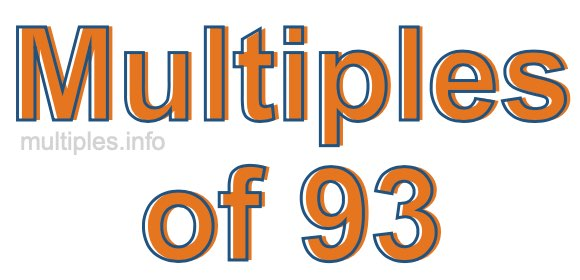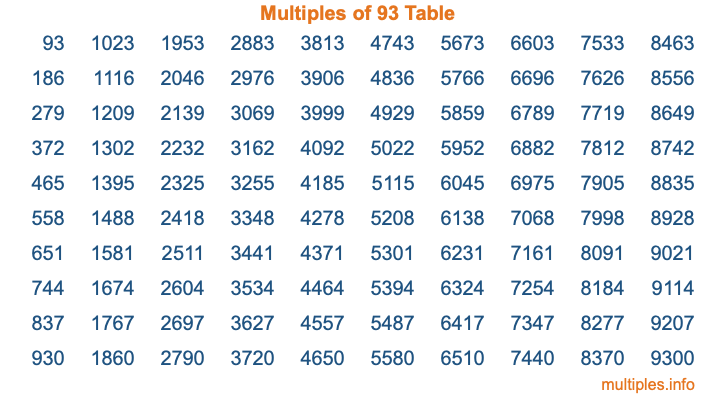Multiples of 93Welcome to the Multiples of 93 page. Here we will first teach you everything you will ever need to know about the multiples of 93, and then give you a study guide summary of everything we taught you to make sure you remember it all. Use this page to look up facts and learn information about the multiples of 93. This page will make you a multiples of ninety-three expert!

Definition of Multiples of 93
Multiples of 93 are all the numbers that when divided by 93 equal an integer. Each of the multiples of 93 are called a multiple. A multiple of 93 is created by multiplying 93 by an integer.

Therefore, to create a list of multiples of 93, you start with 1 multiplied by 93, then 2 multiplied by 93, then 3 multiplied by 93, and so on for as long as you want. Thus, the list of the first five multiples of 93 is 93, 186, 279, 372, and 465. To see a larger list of multiples of 93, see the printable image of Multiples of 93 further down on this page. We also have a category where you can choose any nth multiple of 93.

Multiples of 93 Checker
The Multiples of 93 Checker below checks to see if any number of your choice is a multiple of 93. In other words, it checks to see if there is any number (integer) that when multiplied by 93 will equal your number. To do that, we divide your number by 93. If the the quotient is an integer, then your number is a multiple of 93.

Is  a multiple of 93?

Least Common Multiple of 93 and ...
A Least Common Multiple (LCM) is the lowest multiple that two or more numbers have in common. This is also called the smallest common multiple or lowest common multiple and is useful to know when you are adding our subtracting fractions. Enter one or more numbers below (93 is already entered) to find the LCM.

Check out our LCM Calculator if you need more details about the Least Common Multiple or if you need the LCM for different numbers for adding and subtraction fractions.

nth Multiple of 93
As we stated above, 93 is the first multiple of 93, 186 is the second multiple of 93, 279 is the third multiple of 93, and so on. Enter a number below to find the nth multiple of 93.

th multiple of 93

Multiples of 93 vs Factors of 93
93 is a multiple of 93 and a factor of 93, but that is where the similarities end. All postive multiples of 93 are 93 or greater than 93. All positive factors of 93 are 93 or less than 93.

Below is the beginning list of multiples of 93 and the factors of 93 so you can compare:

Multiples of 93: 93, 186, 279, 372, 465, etc.

Factors of 93: 1, 3, 31, 93

As you can see, the multiples of 93 are all the numbers that you can divide by 93 to get a whole number. The factors of 93, on the other hand, are all the whole numbers that you can multiply by another whole number to get 93.

It's also interesting to note that if a number (x) is a factor of 93, then 93 will also be a multiple of that number (x).

Multiples of 93 vs Divisors of 93
The divisors of 93 are all the integers that 93 can be divided by evenly. Below is a list of the divisors of 93.

Divisors of 93: 1, 3, 31, 93

The interesting thing to note here is that if you take any multiple of 93 and divide it by a divisor of 93, you will see that the quotient is an integer.

Multiples of 93 Table
Below is an image of the first 100 multiples of 93 in a table. The table is in chronological order, column by column. The first column has the first ten multiples of 93, the second column has the next ten multiples of 93, and so on.The Multiples of 93 Table is also referred to as the 93 Times Table or Times Table of 93. You are welcome to print out our table for your studies.

Negative Multiples of 93
Although not often discussed or needed in math, it is worth mentioning that you can make a list of negative multiples of 93 by multiplying 93 by -1, then by -2, then by -3, and so on, to get the following list of negative multiples of 93:

-93, -186, -279, -372, -465, etc.

Multiples of 93 Summary
Below is a summary of important Multiples of 93 facts that we have discussed on this page. To retain the knowledge on this page, we recommend that you read through the summary and explain to yourself or a study partner why they hold true.

There are an infinite number of multiples of 93.

A multiple of 93 divided by 93 will equal a whole number.

93 divided by a factor of 93 equals a divisor of 93.

The nth multiple of 93 is n times 93.

The largest factor of 93 is equal to the first positive multiple of 93.

93 is a multiple of every factor of 93.

93 is a multiple of 93.

A multiple of 93 divided by a divisor of 93 equals an integer.

93 divided by a divisor of 93 equals a factor of 93.

Any integer times 93 will equal a multiple of 93.

Multiples of a Number
Here you can get the multiples of another number, all with the same attention to detail as we did for multiples of 93 on this page.

Multiples of
Multiples of 94
Did you find our page about multiples of ninety-three educational? Do you want more knowledge? Check out the multiples of the next number on our list!